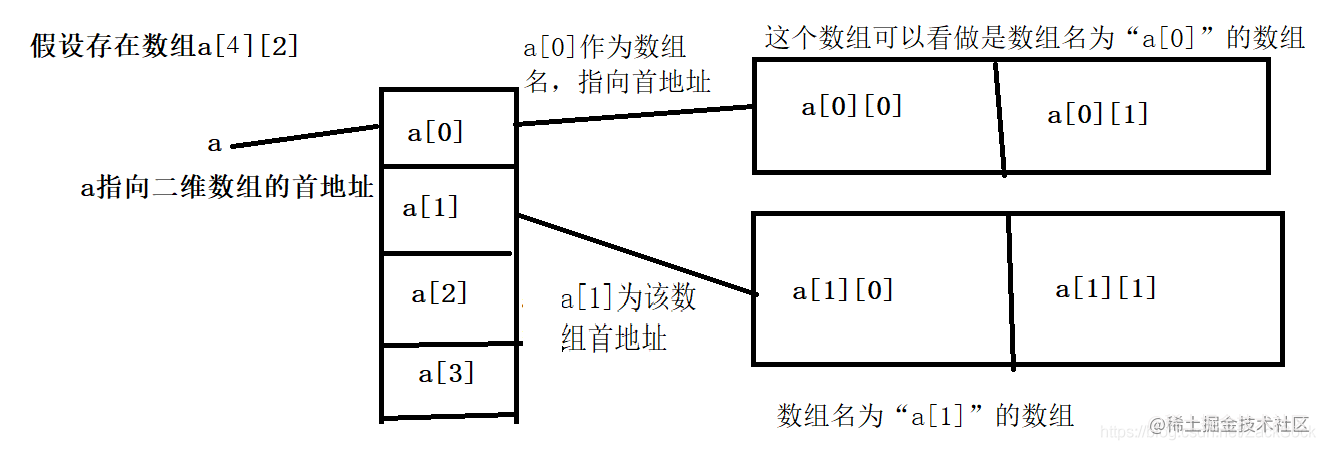# C语言指针

1.1变量和地址

• 变量：直观来说，int a、char ch、float num这些都是声明变量，而a、ch、num就是变量
• 地址：在计算机中，内存被分为一小块一小块的，而每一块都有一个编号，叫做地址。
• 一般变量都存储在内存当中。而每块内存都有一个独一无二的地址，这个地址就是指针
• 如果把内存比作一个宾馆，在声明一个变量时（int a），就相当于在宾馆前台办了入住手续。前台会给你一个门卡和门牌号，简单理解门牌号就是地址。

2.1、指针变量的定义及使用

（1）、指针变量的定义

``````//声明了两个整型变量和一个浮点型变量
int i, j;
float f;

//声明三个指针变量
int *pi, *pj;
float *pf;（2）、指针变量的使用

• 取地址符&：单目运算符 “&” 的功能是取操作对象的地址。常量、表达式和寄存器变量不能取地址，因为它们不是存放在内存某个存储单元中，而是放在寄存器中，寄存器无地址。
• 指针运算符（间接寻址运算符）：单目运算符 **“”** 的功能是按照操作对象的地址值，访问对应存储单元。与“&”互为逆运算。
``````//声明一个变量i，初始化值为10
int i = 10;

//利用取地址符&获取i的地址
printf("%d", &i);

//定义一个指针变量pi，指向i的地址
int *pi = &i;

//利用指针运算符*获取pi指向的内存，即为i的值
printf("%d", *pi);（3）、&和*运算符的结合方向

“&”和“*”两个运算符优先级相同，但按从右至左方向结合。可理解为从右开始运算

``````//声明一个变量i
int i = 10;
//声明一个指针变量pi，指向i
int *pi = &i;
//输出i的地址
printf("%d", &*pi);2.2、指针变量的初始化

``````void main(){
int a = 10;
//利用取地址符&，获取变量a的地址，给指针变量pa赋值
int *pa = &a;
}2.3、指针运算

（1）赋值运算

``````void main(){
int *px, *py, *pz, x;
//1、指向某个地址
px = &x;

//2、赋予空指针
py = NULL;

//3、赋予指定地址
pz = 4000;

}（2）指针与整数的加减运算

• 指针变量自增或自减，即指针向前或者向后移动一个存储单元
• 指针比那里加上一个整型数，即指针向前或者向后移动指定的存储单元

（3）关系运算

• px < py，判断px指向的地址是否小于py指向的地址
• px == py，判断px和py是否指向同一个地址
• px == 0和px != 0表示px是否为空指针

``````//声明函数
void invert(int *a, int start, int end);

/**
*    采用递归法对a数组的元素进行逆序
*/
void main(){

}

/**
*    实现函数
*    a为数组首地址
*    i位起始逆序元素
*    j为逆序结尾元素
*/
void invert(int *a, int start, int end){
//临时变量，用于交换
int temp;

//当起始逆序元素小于逆序结尾元素时，说明还没有逆序到中间元素
if(start < end){
//将起始元素和结尾元素交换
temp = a[start];
a[start] = a[end];
a[end] = temp;

//交换后再次调用invert，将其余元素逆序，此时start和end要同时向中间移动
invert(a, start + 1, end - 1);
}
}3.1、指向数组的指针

• 数组名即为该数组的首地址，a为一个数组，a = &a。
• 可以通过指针对数组元素进行访问，a = a、(a + 1) = a。
• 数组名不能进行指针的操作，像指针p++是合法的，但是数组a++是非法的。

3.2、字符指针和字符数组

（1）字符数组方式

``````void main(){
//定义一个字符数组
char sentence[] = "Do not go gengle into that good night!";
printf("%s", sentence);
}（2）字符指针方式实现字符串

``````void main(){
char *sentence = "Do not go gentle into that good night!";
printf("%s", sentence);
}``````/**
*    用数组将字符串sentence复制到字符串copy
*/
void mian(){
char *sentence = "Do not go gentle into that good night!", copy;
int i;
//当没有遇到结束符时，一直循环
for(i = 0; sentence[i] != '\0'; i++){
//将数据复制到copy中
copy[i] = sentence[i];
}
printf("复制后的copy是：%s", copy);
}3.3、多级指针及指针数组

（1）多级指针

``````void main(){
//定义一个普通变量
int a = 10;

//定义一个指针变量，指向a
int *p = &a;

//定义另一个指针变量，指向指针变量p，此时pp就是二级指针
int **pp = &p;

//输出两个指针
printf("一级指针pa为：%d\n", p);
printf("二级指针ppa为：%d", pp);

//指针的指针和普通指针操作一样，可以用*pp获取pp指向地址中的内容，即p存储的内容
printf("p存储的内容为：%d", *pp);
}（2）指针数组

``````int *a;``````void main(){
//定义并初始化一个int数组
int a = {1, 3, 5, 6, 8}, i;

//定义一个指针数组，与a数组中元素对应
int *p;
for(i = 0; i < 5; i++){
p[i] = &a[i];
}

//定义一个二级指针，存放指针数组的首地址。指针数组和普通数组一样，数组名为数组首地址
int **pp = p;

//利用指针数组首地址输出数据
for(i = 0; i < 5; i++){

//数组a中第零个元素地址为p，而p的的地址为pp，所以**pp = a
//数组a中第一个元素地址为p + 1，而p + 1的地址为pp + 1，所有**(pp + 1) = a
//以此类推，**(pp + n) = a[n]
printf("%d\t", **(pp + i));
}
}3.4、指针与多维数组

（1）多维数组的地址

• 先去掉，只看“a”一个维度。此时a只是个普通的一维数组，而后面的也只是决定了数组a元素的性质
• 在数组a中，有四个元素，我们取一个来分析第二个维度。其第一个元素为a，我们将a看做一个整体，不作为数组元素，只作为一个名称X。那么第二个维度就可以看做X，即一个有两个元素的数组。
• 由上面可知，X数组的首地址为数组名，即X。X实际上是a，类推的话X1、X2等就是a、a。可以间接理解为数组的第一个维度装的全是地址，每个元素X的地址。（2）多维数组的指针

``````void mian(){
//创建一个普通二维数组
int num = {
{1, 3, 4, 5, 6},
{4, 5, 7, 8, 8},
{6, 8, 9, 0, 1},
{3, 4, 2, 1, 2},
{4, 5, 6, 3, 2}
};

//声明一个指针数组
int *p_num;
int i, j;

//初始化指针数组，每个元素分别指向num、num、、、
for(i = 0; i < 5; i++){
p_num[i] = &num[i];
}

//利用指针数组p_num输出num数组中的元素
for(i = 0; i < 5; i++){

printf("这是第%d轮数组\n", i+1);
for(j = 0; j < 5; j++){

//将p_num[i]作为一个数组首地址，数组存储的内容为*(p_num+j)
printf("%d\t", *(p_num[i] + j));
}
printf("\n");
}

}